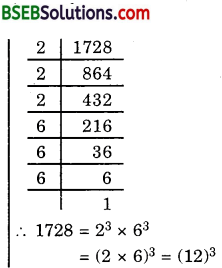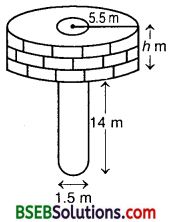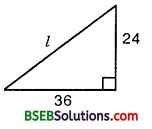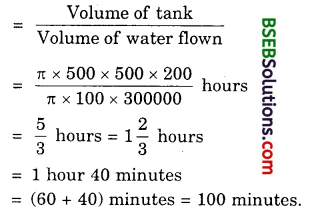# Bihar Board Class 10th Maths Solutions Chapter 13 Surface Areas and Volumes Ex 13.3

Bihar Board Class 10th Maths Solutions Chapter 13 Surface Areas and Volumes Ex 13.3 Textbook Questions and Answers.

## BSEB Bihar Board Class 10th Maths Solutions Chapter 13 Surface Areas and Volumes Ex 13.3Question 1.
A metallic sphere of radius 4.2 cm is melted and recast into the shape of a cylinder of radius 6 cm. Find the height of the cylinder.
Solution:
Volume of the sphere = $$\frac { 4 }{ 3 }$$ πr³
= $$\frac { 4 }{ 3 }$$ x π x (4.2)² cm³
If h is the height of a cylinder of radius 6 cm. Then, its volume
= n(6)²h cm³ = 36πh cm³
Since the volume of metal in the form of sphere and cylinder remains the same, we have
36πh = $$\frac { 4 }{ 3 }$$ x π x 4.2 x 4.2 x 4.2 3
or h = $$\frac { 1 }{ 36 }$$ x $$\frac { 4 }{ 3 }$$ x 4.2 x 4.2 x 42
or h = 2.744
Hence, the height of the cylinder is 2.744 cm.

Question 2.
Metallic spheres of radii 6 cm, 8 cm and 10 cm, respectively, are melted to form a single solid sphere. Find the radius of the resulting sphere.
Solution:
Sum of the volumes of 3 given spheres = $$\frac { 4 }{ 3 }$$π(r1³+r2³+r3³)
= $$\frac { 4 }{ 3 }$$π(6³ + 8³ + 10³) cm³
= $$\frac { 4 }{ 3 }$$π(216 + 512 + 1000) cm³ 3
= $$\frac { 4 }{ 3 }$$π(1728) cm³
Let R be the radius of the new spheres whose volume is the sum of the volumes of the three given spheres.∴ $$\frac { 4 }{ 3 }$$πr³ = $$\frac { 4 }{ 3 }$$π(1728)
or R³ = 1728
or R³ = (12)³
or R = 12
Hence, the radius of the resulting sphere is 12 cm.Question 3.
A 20 m deep well with diameter 7 m is dug and the earth from digging is evenly spread out to form a platform 22 m by 14 m. Find the height of the platform.
Solution:
Let h m be the required height of the platform.
The shape of the platform will be like the shape of a cuboid of dimensions 22 m x 14 m x h m.
The volume of the platform will be equal to the volume of the earth dug out from the well.
Now, the volume of the earth
= Volume of the cylindrical well
= πr²h = $$\frac { 22 }{ 7 }$$ x 12.25 x 20 m³ [∵ r = $$\frac { 7 }{ 2 }$$ m = 3.5 m]
= 770 m³
Also, the volume of the platform = 22 x 14 x h m³
But volume of the platform – Volume of the well
i.e., 22 x 14 x h = 770
h = $$\frac { 770 }{ 22×14 }$$
Height of the platform = 2.5 m.

Question 4.
A well of diameter 3 m is dug 14 m deep. The earth taken out of it has been spread evenly all around it in the shape of a circular ring of width 4 m to form an embankment. Find the height of the embankment.
Solution:
Let h be the required height of the embankment.The shape of the embankment will be like the shape of a cylindrical shell of internal radius 1.5 m and external radius (4 + 1.5) m = 5.5 m (see figure).
The volume of the embankment will be equal to the volume of earth dug out from the well.
Now, the volume of the earth
= Volume of the cylindrical well
= π x (1.5)² x 14 m³ = 31.5π m³
Also, the volume of the embankment
= π(5.5² – 1.52)h m³
= π(5.5 + 1.5 x 5.5 – 1.5)h m³
= π x 7 x 4h m³
= 28πh m³
Hence, we have :
28πh = 31.5π
So h = $$\frac { 31.5 }{ 28 }$$ = 1.125
Hence, the required height of the embankment is 1.125 m.Question 5.
A container shaped like a right circular cylinder having diameter 12 cm and height 15 cm is full of ice cream. The ice cream is to be filled into cones of height 12 cm and diameter 6 cm, having hemispherical shape on the top. Find the number of such cones which can be filled with ice cream. [Annual Paper (Delhi) 2008]
Solution:
Volume of the cylinderVolume of a cone having hemispherical shape on the top
= $$\frac { 1 }{ 3 }$$πr²h + $$\frac { 2 }{ 3 }$$πr³ = $$\frac { 1 }{ 3 }$$ πr²(h + 2r)
= $$\frac { 1 }{ 3 }$$π($$\frac { 6 }{ 2 }$$)² (12 + 2 x $$\frac { 6 }{ 2 }$$) cm³
= $$\frac { 1 }{ 3 }$$π x 3² x 18 cm³
Let the number of cone that can be filled with ice cream
Then, $$\frac { 1 }{ 3 }$$π x 32 x 18 x n = π x 6² x 15
n = $$\frac{\pi \times 6 \times 6 \times 15}{\pi \times 3 \times 3 \times 18}$$ x 3 = 10

Question 6.
How many silver coins, 1.75 cm in diameter and of thickness 2 mm, must be melted to form a cuboid of dimensions 5.5 cm x 10 cm x 3.5 cm?
Solution:
The shape of the coin will be like the shape of a cylinder of radius $$\frac { 1.75 }{ 2 }$$ cm
0.875 cm and of height 2 mm = $$\frac { 2 }{ 10 }$$ cm = 0.2 cm.
Its volume
= πr²h
= $$\frac { 22 }{ 7 }$$ x 0.875 x 0.875 x 0.2 cm³
= 0.48125 cm³
Volume of the cuboid
= 5.5 x 10 x 3.5 cm³ = 192.5 cm³
Number of coins required to form the cuboid
= $$\frac{\text { Volume of the cuboid }}{\text { Volume of a coin }}$$
= $$\frac{192.5}{0.48125}$$
= 400
Volume of a coin 0.48125
Hence, 400 coins must be melted to form a cuboid of required dimensions.Question 7.
A cylindrical bucket, 32 cm high and with radius of base 18 cm, is filled with sand. The bucket is emptied on the ground and a conical heap of sand is formed. If the height of the conical heap is 24 cm, find the radius and slant height of the heap.
Solution:
Volume of the sand = Volume of the cylindrical bucket
= πr²h = π x 18 x 18 x 32 cm³
Volume of the conical heap= $$\frac { 1 }{ 3 }$$πr²h, where r = ?, h = 24 cm
= $$\frac { 1 }{ 3 }$$πr2 x 24 cm³ = 8πr²
The volume of the conical heap will be equal to that of sand.
∴ 8πr² = π x 18 x 18 x 32
or r² = 18 x 18 x 4 = 18² x 2²
or r = 18 x 2 = 36
Here, slant height l = $$\sqrt{r^{2}+h^{2}}$$
So, l = $$\sqrt{36^{2}+24^{2}}$$
= $$\sqrt{12 \times 12(3 \times 3+2 \times 2)}$$
= 12$$\sqrt{9+4}$$ = 12$$\sqrt{13}$$
Hence, the radius of the conical heap is 36 cm and its slant height is 12$$\sqrt{13}$$ cm.

Question 8.
Water in a canal 6 m wide and 1.5 m deep, is flowing with a speed of 10 km/h. How much area will it irrigate in 30 minutes, if 8 cm of standing water is needed ?
Solution:
Width of the canal = 6 m
Depth of the canal = 1.5 m
Length of water column per hour = 10 km
Length of water column in 30 minutes or $$\frac { 1 }{ 2 }$$ hours
= $$\frac { 1 }{ 2 }$$ x 10 km = 5000 m
Volume of water flown in 30 minutes
= 1.5 x 6 x 5000 m³ = 45000 m³
Now, 8 cm = $$\frac { 8 }{ 100 }$$ m
i.e., 0.08 m standing water is desired.
∴ Area irrigated in 30 minutes
= $$\frac{\text { Volume }}{\text { Height }}$$
= $$\frac{45000}{0.08}$$
= 562500 m²
or 56.25 hectaresQuestion 9.
A farmer connects a pipe of internal diameter 20 cm from a canal into a cylindrical tank in his field, which is 10 m in diameter and 2 m deep. If water flows through the pipe at the rate of 3 km/h, in how much time will the tank be filled?
Solution:
Diameter of the pipe = 20 cm
So, Radius of the pipe = 10 cm
Length of water column per hour = 3 km
= 3 x 1000 x 100 cm
= 300000 cm
Volume of water flown in one hour = π x 100 x 300000 cm³
Tank to be filled = Volume of cylinder (with r = 5 m = 500 cm and h = 2 m = 200 cm)
= π x 500 x 500 x 200 cm³
Time required to fill the tank